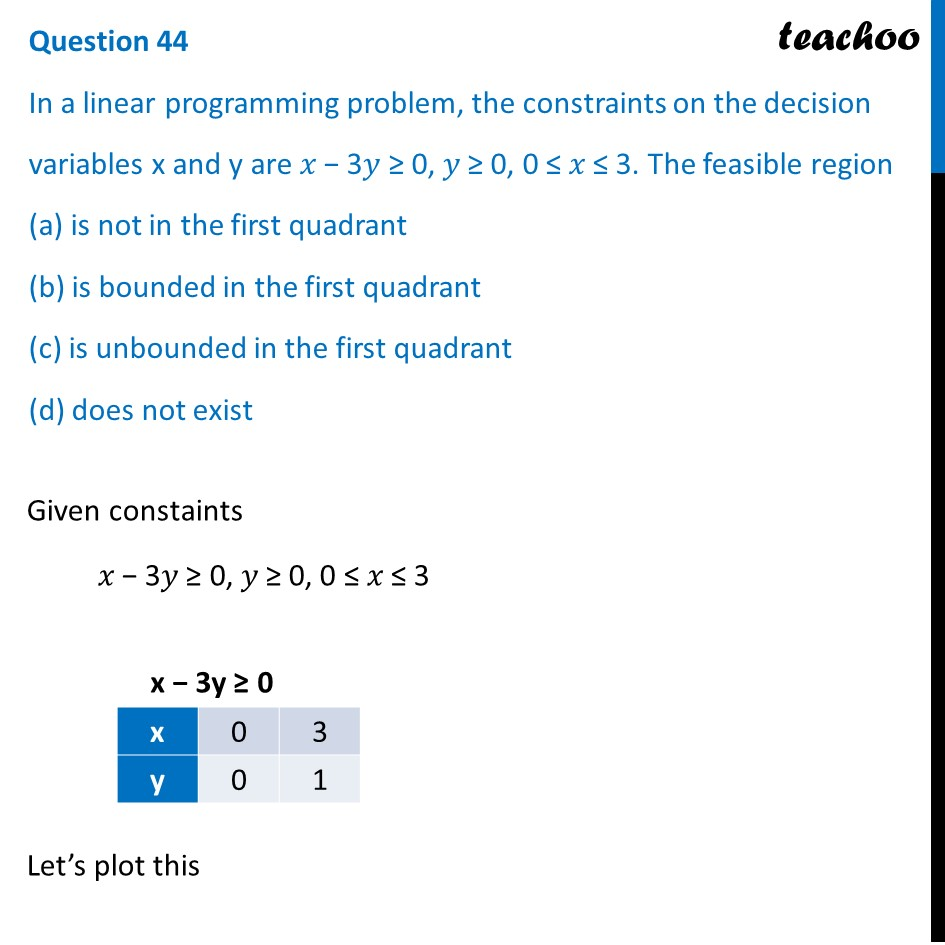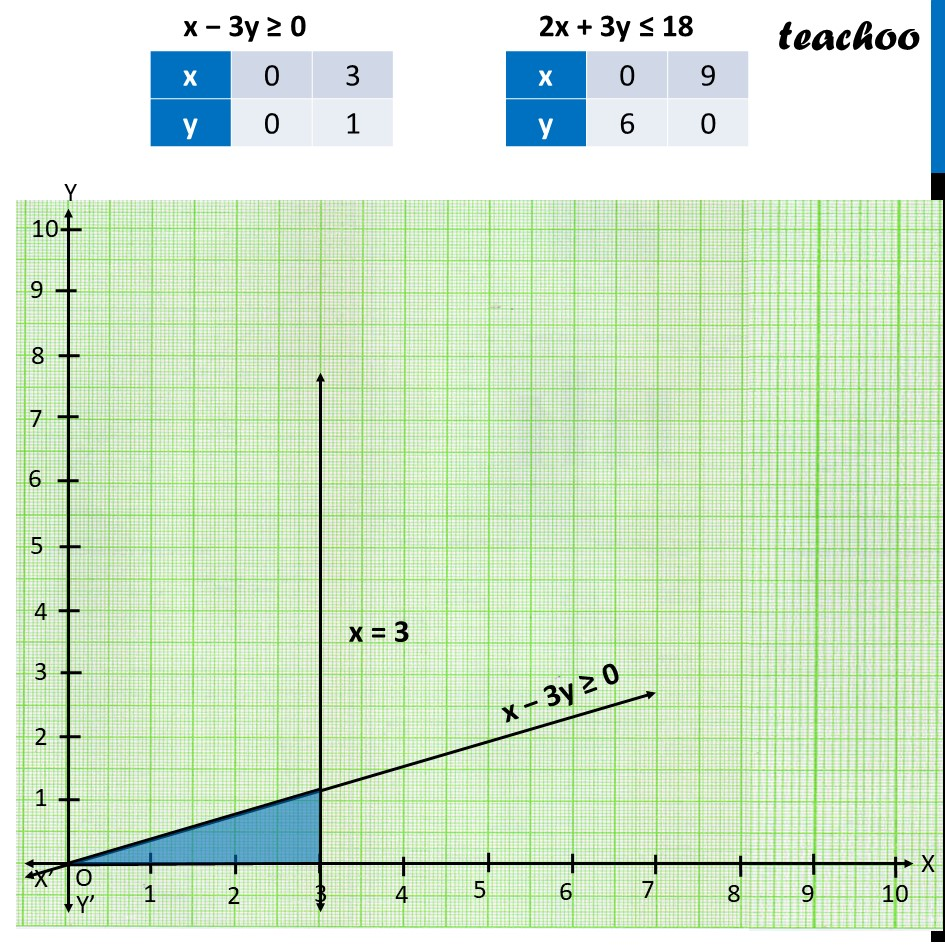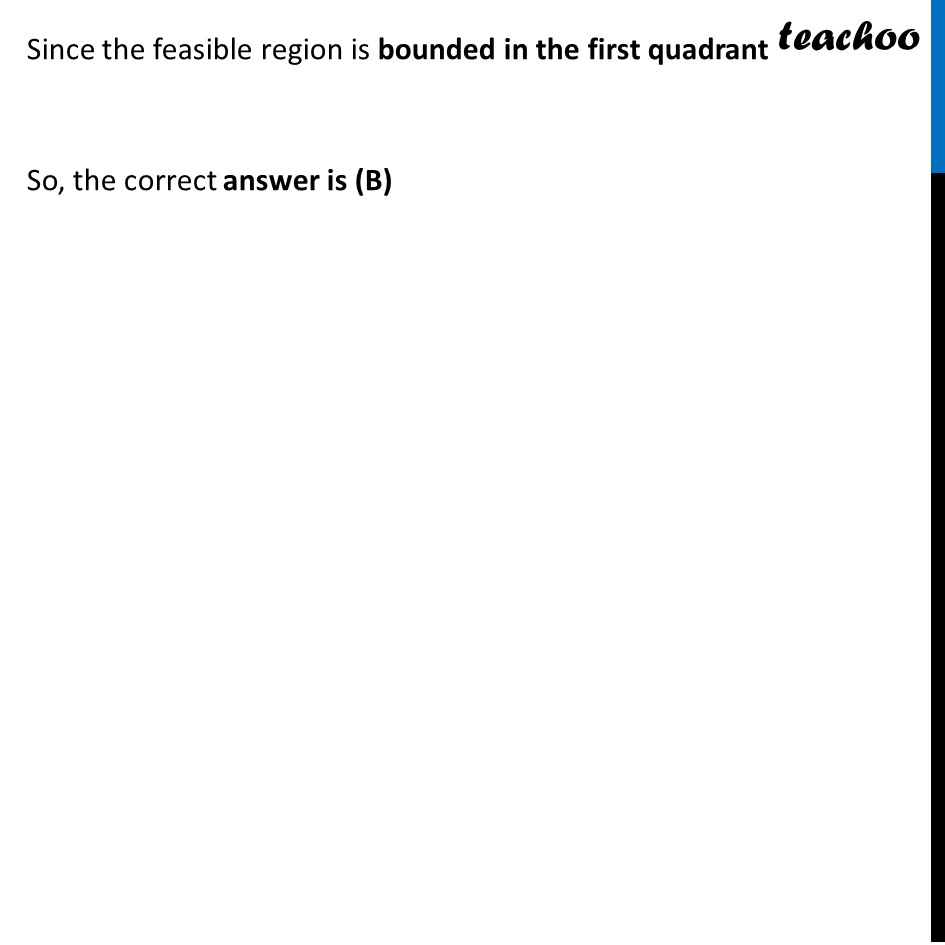CBSE Class 12 Sample Paper for 2022 Boards (MCQ Based - for Term 1)

Class 12
Solutions of Sample Papers and Past Year Papers - for Class 12 Boards

##(adsbygoogle = window.adsbygoogle || []).push({});(adsbygoogle = window.adsbygoogle || []).push({});(adsbygoogle = window.adsbygoogle || []).push({});

### Transcript

Question 44 In a linear programming problem, the constraints on the decision variables x and y are 𝑥 − 3𝑦 ≥ 0, 𝑦 ≥ 0, 0 ≤ 𝑥 ≤ 3. The feasible region (a) is not in the first quadrant (b) is bounded in the first quadrant (c) is unbounded in the first quadrant (d) does not exist Given constaints 𝑥 − 3𝑦 ≥ 0, 𝑦 ≥ 0, 0 ≤ 𝑥 ≤ 3 Let’s plot this Since the feasible region is bounded in the first quadrant So, the correct answer is (B)